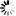# Publications in area "Mathematical Modeling & Simulation"

All || By Area || By Year

Title Published SHARCNET Authors Area
1. 2012 Pawel Pralat Random Graph Theory Mathematical Modeling & Simulation
2. 2010 S.Gaspers, M.E.Messinger, R.J.Nowakowski, and P.Pralat Random Graph Theory Mathematical Modeling & Simulation
3. 2010 P.Gordinowicz and P.Pralat Random Graph Theory Mathematical Modeling & Simulation
4. 2010 R. Angelova, M. Lipczak, E. Milios, and P. Pralat Random Graph Theory Mathematical Modeling & Simulation
5. 2008 P.Pralat Mathematical Modeling & Simulation
6. 2008 J. KaupuÅ¾s, R.V.N. Melnik, J.RimÅ¡Äns Mathematical Modeling & Simulation
7. 2008 R. Angelova, M. Lipczak, E. Milios, and P. Pralat Mathematical Modeling & Simulation
8. 2008 Pawel Pralat Random Graph Theory Mathematical Modeling & Simulation
9. 2008 A.T. Lawniczak Mathematical Modeling & Simulation
10. 2008 Pawel Pralat Random Graph Theory Mathematical Modeling & Simulation
11. 2008 R. Angelova, M. Lipczak, E. Milios, and P. Pralat Mathematical Modeling & Simulation
12. 2008 R. Angelova, M. Lipczak, E. Milios, P. Pralat Random Graph Theory Mathematical Modeling & Simulation
13. 2008 S. Xie, A.T. Lawniczak and P. LiÏ Mathematical Modeling & Simulation
14. 2008 A.T. Lawniczak and S. Xie Mathematical Modeling & Simulation
15. 2008 R. Amariei, A.R. Willms, C.T. Bauch Mathematical Modeling & Simulation
16. 2008 Kohler, Timothy A., Mark D. Varien, Aaron M. Wright, and Kristin K. Kuckelman Mathematical Modeling & Simulation
17. 2008 3. P. LiÏ, A.T. Lawniczak, S. Xie and J. Xu Mathematical Modeling & Simulation
18. 2007 Reza Dorrigiv, Alejandro Lopez-Ortiz, Pawel Pralat Random Graph Theory Mathematical Modeling & Simulation
19. 2007 C.T. Bauch, A.S.R.S Rao, B. Pham, M Krahn, V. Gilca, B. Duval, M.H. Chen, A Tricco Mathematical Modeling & Simulation
20. 2007 J. KaupuÅ¾s, R. V. N. Melnik, J. Rimshans Mathematical Modeling & Simulation
Back to all areas